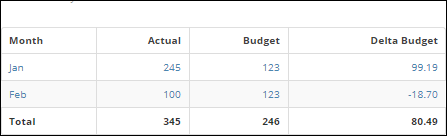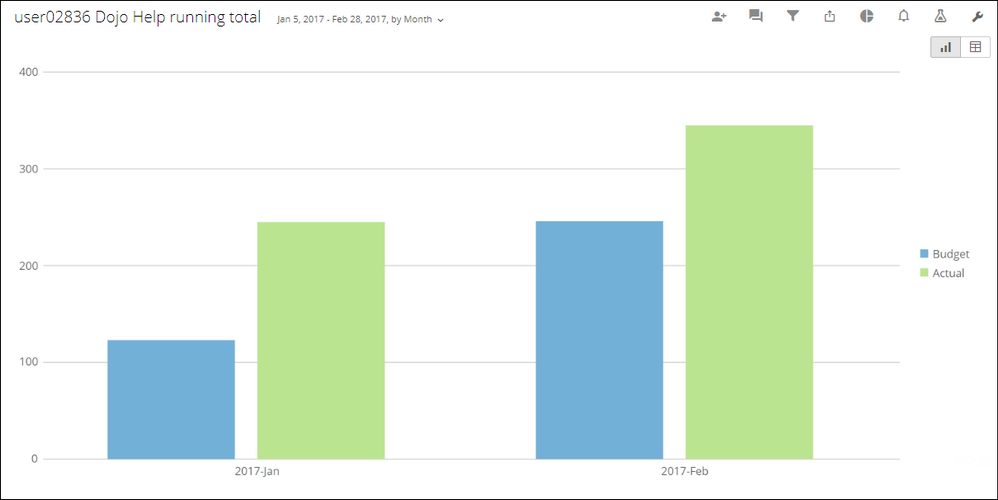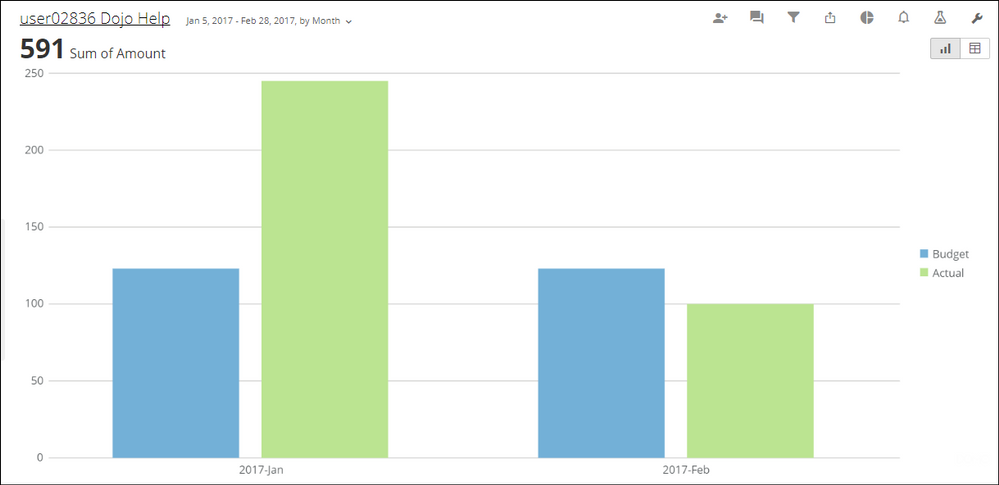# How to add a calculation to beast mode that involves uncollapsing columns into rows

I have a Data table with the Actual amounts for Accounts Receivables to which I have appended another table that has the budget amounts on a monthly basis. I created a sumo card to view the data in pivot format . I now need to add a calculation to the sumo card, but the calculation involves data that comes from the same column.

I assumed that I would have to uncollapse this column in MAgic ETL and then add a calculation as a separate column so that I can view it in a sumo card. But this didn't work

I have attached an excel sheet to explain the dataset I have and what I currenrly see in Sumo View, and what I actually want to see.

Can Someone guide me through this? I Know it should be simple but I am clearly missing some logic here.

I will also include a screenshot of what I tried in Magic ETL

• I struggled with this one.  This may be a case where Excel performs better than Domo at the moment.  I could not find a way to get the calculated `Delta Budget` field to calculate currectly for the total row.  Instead, you have to pick an agregate function (sum, min, max, avg, etc.) which does not work at all.You can see in the above screenshot, it simply summed the delta budget field.  It would be nice if the Total Row would allow the beast mode calculation to be performed on the other totals in the row.  However, I do not believe that is possible in Domo right now.

You can build some other visuals, unless you are tied to the sumo card / pivot table look:• Hi Scott,

I tried the same method that you did and you're right I had to pick an aggregate which did not give me the right answers. I need this to be in the sumo view as it gives me the closest match to the way the data needs to be presented. But thankyou for helping. ?